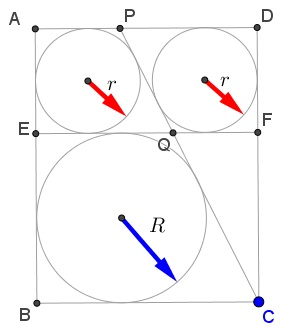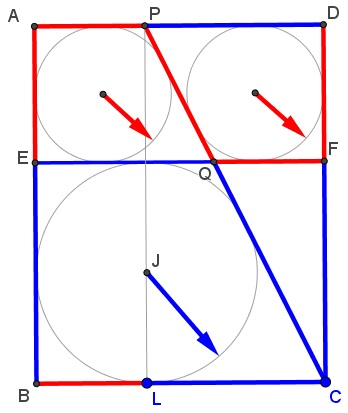# A Modified Sangaku

### Source### Problem

In rectangle $ABCD,$ $E\in AB,$ $F\in CD,$ $EF\parallel BC;$ $P\in AD;$ $Q=CP\cap EF.$

Each of the quadrilaterals $AEQP,$ $DPQF,$ and $BCQE$ is inscriptible, the former two are equal.If $r$ is the common radius of the incircles of $AEQP$ and $DPQF,$ and $R$ the inradius of $BCQE,$ prove that $\displaystyle \frac{R}{r}=\varphi,$ the Golden Ratio.

### Solution

Extend $CP$ and $BA$ to the cross-point $K:Obviously, line$PQ$passes through the center of the rectangle$AEFD.$That point projects to the midpoint of$BC,$implying that it is also the midpoint of$KC.$Thus,$BE=AK=2R$and$AE=2r.$The incircle of$BCQE$serves as the incircle of$\Delta BCK$while the incircle of$AEQP$is the incircle of$\Delta EQK.$We thus have the proportion:$\displaystyle \frac{BK}{EK}=\frac{4R+2r}{2R+2r}=\frac{R}{r},$giving an equality$\displaystyle (2R+r)r=(R+r)R,$or,$R^2-Rr-r^2=0,$from which$\displaystyle \frac{R}{r}=\frac{\sqrt{5}+1}{2}.\$

### Extra

As the consequence of the above, it is easily seen that the configuration sports multiple occurrences of the Golden Ratio, as is illustrated below.### Acknowledgment

The problem has been posted by Kadir Altintas at the Peru Geometrico facebook group, with a reference to Géry Huvent note Sangaku.

Several additional solutions can be found by following the link above to the Peru Geometrico.